Singlelayer air core coil winding formulas - 3.7 out of 5 based on 7 votes
Rating 3.71 (7 Votes)

Singlelayer air core coil winding formulas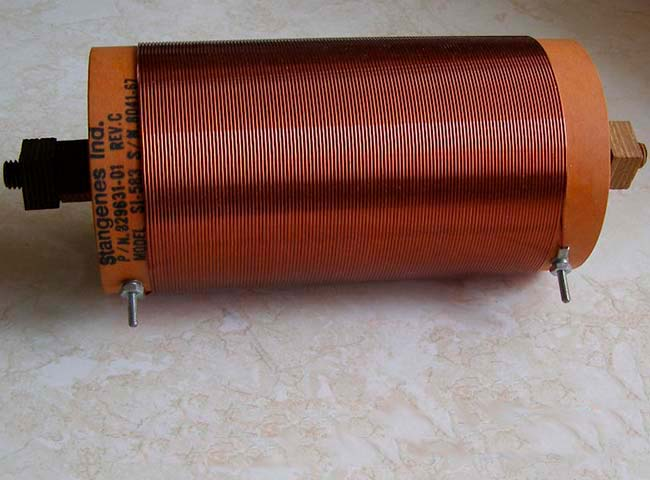Single-layer inductor is a wire rolled into a helix. For adding stiffness the wire is usually wound on a cylindrical pipe named coil-former. Therefore the initial parameters in Coil32 are the dimensions of the coil-former and the diameter of the wire, because they are easier to measure in practice. However, the calculating formulas use the geometrical parameters of the helix.

Single-layer coil is widespread, especially for designs at shortwave and medium wave Amateur radio bands. The basic goodies of single-layer coils is high Q-factor, relatively small parasitic self-capacitance, ease of manufacturing. Consider the methods of calculation of the one-layer inductor without a gap between the turns , ie close-winding.Historically, the first who derived a formula for calculating such inductor was J.C.Maxwell. He used a model in which a separate turn were presented in the form of a circular loop of infinitely thin wire, ie coaxial circular filaments model. H.A.Lorenz used the model of an infinitely long solenoid in which a helix was presented as infinitely thin conducting tape with no gap between turns as on a figure, ie current sheet model. His formula has a very good approximation for round wire coils if they have many turns and the windings are closely spaced.

In 1909, the Japanese physicist H. Nagaoka rearranged the formula of Lorentz to form which indicated an important conclusion is that the inductance of a solenoid depends only on the shape and size of the helix. Formula Nagaoka can be written as: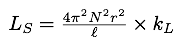• Ls - inductance of the coil
• N - number of turns of the coil
• r - radius of the winding
• l - length of the winding
• kL - Nagaoka's coefficient

The most important conclusion from the analysis of this formula was that the Nagaoka coefficient depended only on the ratio l/D, which was called the form factor of the coil.

For the long solenoid this formula seems as following: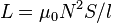where S is the cross-sectional area and l is the length of the solenoid. This formula is only of academic interest and is not suitable for calculations of real coils, since only valid for an almost infinitely long solenoid, which does not exist in practice.

Single-layer air core inductor can be calculated by the numerical method, using the Maxwell's o Nagaoka's formula for the solenoid. However, modern more simple empirical formulas give very high accuracy of the calculations and is quite sufficient for practical purposes.

Review and selection of empirical formulas begin with the most famous H.A.Wheeler's formula. Usually this formula is often used in various programs, online calculators, hand books and articles devoted to the calculation of inductance. This formula looks like this:

 L = a2 N2 / ( 9 a + 10 b ) 

where N is the number of turns, a and b are respectively the radius and the length of the winding. Dimension in inches. Adapting this formula for the metric system (rather CGS system of units) and changing the radius to the diameter, we get the following: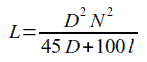• L - inductance [µH]
• N- number of turns of the inductor [cm]
• D - diameter of the winding [cm]
• l - length of the winding [cm]

The formula was proposed by Wheeler in the distant 1928, when people could only dream of computers and it was very useful at that time because allowed "in a column" on a piece of paper to calculate practical inductor. The formula got root in the mass consciousness of radiohams. However, few people know that this formula, just like any empirical formula, has limitations. This formula gives an error of up to 1% when l/D > 0,4, i.e. if the coil is not too short. For short coils this formula is not suitable.

Was followed by several attempts to remedy this deficiency. In 1985, R. Lundin has published two empirical formulas, one for short coils and one for long coils, allowing to calculate the Nagaoka coefficient with an error not bigger than 3ppM (±0.0003%), which is undoubtedly higher precision then precision of manufacturing or measuring the inductance of the coil. Here is a calculator based on these formulas.

In 1982, 54 years later, with the advent of computers, Wheeler published his "long" formula that calculate a single-layer inductor with an error less than ±0.1%, both long and short coils. In the future, this formula has been improved by R. Rosenbaum, and later by R. Weaver - analysis and the derivation of the formula on R. Weaver's website [ref. 1 ⬇].• Dk - diameter of the winding [mm]
• N - number of turns
• k = l/Dk - form-factor of the coil, the ratio of winding length to its diameter

As a result, we have a formula that allows to compute the single-layer coil with an error less than 18.5 ppM (compared to formula Nagaoka), which is worse than the Lundine's formulas, but firstly it is more than enough for practical calculations, and secondly we have a single more simple formula instead of two for calculating a single-layer inductor, regardless of its form factor. Formula  used in the online calculator of onelayer air core inductor, in older versions Coil32, as well as in all versions of the software for Linux and Android application. Special thanks to R.Weaver for help.

The Coil32  for Windows, cross-platform app Coil64  and version for Android use a more complex method of calculation of the single-layer coil, taking into account the helix form of turns and an arbitrary pitch of winding. Note, that the real inductor always have a helical winding and nonzero gap between turns.

In 1907 E.Roza comparing calculations by the method of Maxwell and Lorentz deduced table of corrections that significantly increases the accuracy of the calculation by Lorentz's method, especially if the coil has a small number of turns. [ref. 2 ⬇] The Rosa's corrections takes into accout that real wire have a round cross-section and so it was named Round Wire Corrections. In 2008, R. Weaver has created an empirical algorithm that allows to calculate the Round Wire Corrections by the numerical method without using tables [ref. 3 ⬇]. This algorithm is applied in Coil32, online calculator, as well as in versions Coil32 for Android and Linux, to increase the accuracy of the calculation of the inductance of single-layer air core inductor.

In addition to inductance, that is the primary parameter of a single-layer coil, the Coil32 calculates other parameters of an inductor:

This data is sufficient to create a realistic model of the coil in the circuit design simulation programs. For example,  in a popular free LTSpice IV.

Moreover, you can calculate the additional results for the parallel resonant circuit at the operating frequency. The characteristic resistance of the LC-circuit is equal to the coil reactance at the resonance frequency.

References:

Сomments from anonymous guests are enabled with moderation.

Coil Winding Machine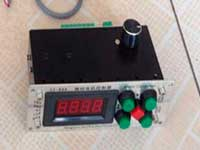US \$187.53
The system consists of individual components including a controller, a stepping motor, a foot switch, power adapter and output shaft. This design makes it very easy for you to integrate the system into your own project.

LC Meter LC200A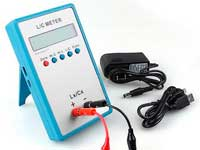US \$37.99
Measuring Capacitance Range:
0.01 pF - 100mF
Measuring Inductance Range:
0.001 uH - 100H
Measurement accuracy:1%.

RF Inductors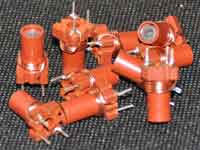US \$6.43
Variable Ferrite Core Inductor coil set 3.5Turns 25-100MHZ

Auto Ranging LCR Meter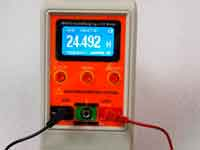US \$63.07
Meter can measure 0.00pF to 100.00mF capacitance, 0.000uH to 100.00H inductance and 0.00? to 10.000M? resistance. Very suitable for small value capacitor and inductor measurement.